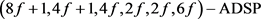# 8阶分圆类构造几乎差集偶Constructions of Almost Difference Set Pairs by Cyclotomic Classes of Order Eight

DOI: 10.12677/AAM.2020.92020, PDF, HTML, XML, 下载: 172  浏览: 262

Abstract: Almost difference set pairs are widely used in many problems because they are closely related to almost optimal binary sequence pairs. Cyclotomic classes method is an important method to con-struct almost difference set pairs. In this paper, several new almost difference set pairs are con-structed by using cyclotomic classes of order eight.

1. 引言

2. 基础知识

${Z}_{q}^{*}={\cup }_{i=0}^{e-1}{C}_{i}$

3. 8阶分圆类构造几乎差集偶

1) 当f为偶数且2不是4次剩余，且 $b=-y,x-a=4$ 时，

1、 $\left(U,W\right)$ 构成 $\left(8f+1,4f,4f,2f,2f-1,2f\right)-\text{ADSP}$Table 1. Relations of cyclotomic numbers of order 8 for even fTable 2. The fifteen basic cyclotomic numbers of order 8 when f is even and 2 is not a quartic residue

2、 $\left({U}^{\prime },W\right)$ 构成 $\left(8f+1,4f+1,4f,2f,2f,6f\right)-\text{ADSP}$

3、 $\left(U,{W}^{\prime }\right)$ 构成 $\left(8f+1,4f,4f+1,2f,2f,6f\right)-\text{ADSP}$

4、 $\left({U}^{\prime },{W}^{\prime }\right)$ 构成 $\left(8f+1,4f,4f+1,2f+1,2f,2f\right)-\text{ADSP}$

2) 当f为偶数且2不是4次剩余，且 $b=4-y,x-a=4$ 时， $\left(U,W\right)$ 构成 $\left(8f+1,4f,4f,2f,2f-1,2f\right)-\text{ADSP}$

$\begin{array}{c}{\text{Δ}}_{i}=|\left({C}_{0}+{\theta }^{i}\right)\cap {C}_{0}|+|\left({C}_{0}+{\theta }^{i}\right)\cap {C}_{4}|+|\left({C}_{0}+{\theta }^{i}\right)\cap {C}_{5}|+|\left({C}_{0}+{\theta }^{i}\right)\cap {C}_{6}|\\ \text{\hspace{0.17em}}\text{\hspace{0.17em}}+|\left({C}_{1}+{\theta }^{i}\right)\cap {C}_{0}|+|\left({C}_{1}+{\theta }^{i}\right)\cap {C}_{4}|+|\left({C}_{1}+{\theta }^{i}\right)\cap {C}_{5}|+|\left({C}_{1}+{\theta }^{i}\right)\cap {C}_{6}|\\ \text{\hspace{0.17em}}\text{\hspace{0.17em}}+|\left({C}_{2}+{\theta }^{i}\right)\cap {C}_{0}|+|\left({C}_{2}+{\theta }^{i}\right)\cap {C}_{4}|+|\left({C}_{2}+{\theta }^{i}\right)\cap {C}_{5}|+|\left({C}_{2}+{\theta }^{i}\right)\cap {C}_{6}|\\ \text{\hspace{0.17em}}\text{\hspace{0.17em}}+|\left({C}_{4}+{\theta }^{i}\right)\cap {C}_{0}|+|\left({C}_{4}+{\theta }^{i}\right)\cap {C}_{4}|+|\left({C}_{4}+{\theta }^{i}\right)\cap {C}_{5}|+|\left({C}_{4}+{\theta }^{i}\right)\cap {C}_{6}|\end{array}$

$\begin{array}{l}=\left(-i,-i\right)+\left(-i,4-i\right)+\left(-i,5-i\right)+\left(-i,6-i\right)+\left(1-i,-i\right)+\left(1-i,4-i\right)\\ \text{\hspace{0.17em}}\text{\hspace{0.17em}}\text{ }\text{ }+\left(1-i,6-i\right)+\left(1-i,5-i\right)+\left(2-i,-i\right)+\left(2-i,4-i\right)+\left(2-i,5-i\right)\\ \text{\hspace{0.17em}}\text{\hspace{0.17em}}\text{ }\text{ }+\left(2-i,6-i\right)+\left(4-i,-i\right)+\left(4-i,4-i\right)+\left(4-i,5-i\right)+\left(4-i,6-i\right)\end{array}$

${\Delta }_{0}={\Delta }_{4}=\frac{16q-4x+16y+4a+16b-64}{64}$

${\Delta }_{1}={\Delta }_{5}={\Delta }_{2}={\Delta }_{6}=\frac{16q+4x-4a-32}{64}$

${\Delta }_{3}={\Delta }_{7}=\frac{16q-4x-16y+4a-16b}{64}$

${\Delta }_{0}={\Delta }_{1},|{\Delta }_{1}-{\Delta }_{3}|=1$，即要满足

$\frac{16q-4x+16y+4a+16b-64}{64}=\frac{16q+4x-4a-32}{64}$

$|\frac{16q+4x-4a-32}{64}-\frac{16q-4x-16y+4a-16b}{64}|=1$

${\Delta }_{0}={\Delta }_{3},|{\Delta }_{1}-{\Delta }_{3}|=1$，即

$\frac{16q-4x+16y+4a+16b-64}{64}=\frac{16q-4x-16y+4a-16b}{64}$

$|\frac{16q+4x-4a-32}{64}-\frac{16q-4x-16y+4a-16b}{64}|=1$

${\Delta }_{1}={\Delta }_{3},|{\Delta }_{0}-{\Delta }_{1}|=1$，即

$\frac{16q+4x-4a-32}{64}=\frac{16q-4x-16y+4a-16b}{64}$

$|\frac{16q-4x+16y+4a+16b-64}{64}-\frac{16q+4x-4a-32}{64}|=1$

1) 当f为偶数且2不是4次剩余，且 $b=-y,x-a=4$ 时，

1、 $\left(U,W\right)$ 构成 $\left(8f+1,4f,4f,2f,2f-1,2f\right)-\text{ADSP}$

2、 $\left({U}^{\prime },W\right)$ 构成 $\left(8f+1,4f+1,4f,2f,2f,6f\right)-\text{ADSP}$

3、 $\left(U,{W}^{\prime }\right)$ 构成 $\left(8f+1,4f,4f+1,2f,2f,6f\right)-\text{ADSP}$

4、 $\left({U}^{\prime },{W}^{\prime }\right)$ 构成 $\left(8f+1,4f+1,4f+1,2f+1,2f,2f\right)-\text{ADSP}$

2) 当f为偶数且2不是4次剩余，且 $b=4-y,x-a=4$ 时， $\left({U}^{\prime },{W}^{\prime }\right)$ 构成 $\left(8f+1,4f+1,4f+1,2f+1,2f,2f\right)-\text{ADSP}$

1) 当f为偶数且2不是4次剩余，且 $b=-y,x-a=4$ 时，

1、 $\left(U,W\right)$ 构成 $\left(8f+1,4f,4f,2f,2f-1,2f\right)-\text{ADSP}$

2、 $\left({U}^{\prime },W\right)$ 构成 $\left(8f+1,4f+1,4f,2f,2f,6f\right)-\text{ADSP}$

3、 $\left(U,{W}^{\prime }\right)$ 构成 $\left(8f+1,4f,4f+1,2f,2f,6f\right)-\text{ADSP}$

4、 $\left({U}^{\prime },{W}^{\prime }\right)$ 构成 $\left(8f+1,4f+1,4f+1,2f+1,2f,2f\right)-\text{ADSP}$

2) 当f为偶数且2不是4次剩余，且 $b=4-y,x-a=4$ 时， $\left({U}^{\prime },W\right)$ 构成 $\left(8f+1,4f+1,4f,2f,2f,6f\right)-\text{ADSP}$

1) 当f为偶数且2不是4次剩余，且 $b=y,x-a=4$ 时，

1、 $\left(U,W\right)$ 构成 $\left(8f+1,4f,4f,2f,2f-1,2f\right)-\text{ADSP}$

2、 $\left({U}^{\prime },W\right)$ 构成 $\left(8f+1,4f+1,4f,2f,2f,6f\right)-\text{ADSP}$

3、 $\left(U,{W}^{\prime }\right)$ 构成 $\left(8f+1,4f,4f+1,2f,2f,6f\right)-\text{ADSP}$

4、 $\left({U}^{\prime },{W}^{\prime }\right)$ 构成 $\left(8f+1,4f+1,4f+1,2f+1,2f,2f\right)-\text{ADSP}$

2) 当f为偶数且2不是4次剩余类，且 $b=y-4,x-a=4$ 时， $\left({U}^{\prime },W\right)$ 构成1) 当f为偶数且2不是4次剩余， $b=y,x-a=4$ 时，

1、 $\left(U,W\right)$ 构成 $\left(8f+1,4f,4f,2f,2f-1,2f\right)-\text{ADSP}$

2、 $\left({U}^{\prime },W\right)$ 构成 $\left(8f+1,4f+1,4f,2f,2f,6f\right)-\text{ADSP}$

3、 $\left(U,{W}^{\prime }\right)$ 构成 $\left(8f+1,4f,4f+1,2f,2f,6f\right)-\text{ADSP}$

4、 $\left({U}^{\prime },{W}^{\prime }\right)$ 构成 $\left(8f+1,4f+1,4f+1,2f+1,2f,2f\right)-\text{ADSP}$

2) 当f为偶数且2不是4次剩余，且 $b=y-4,x-a=4$ 时， $\left(U,W\right)$ 构成 $\left(8f+1,4f,4f,2f,2f-1,2f\right)-\text{ADSP}$

1) 当f为偶数且2不是4次剩余，且 $b=y,x-a=4$ 时，

1、 $\left(U,W\right)$ 构成 $\left(8f+1,4f,4f,2f,2f-1,2f\right)-\text{ADSP}$

2、 $\left({U}^{\prime },W\right)$ 构成 $\left(8f+1,4f+1,4f,2f,2f,6f\right)-\text{ADSP}$

3、 $\left(U,{W}^{\prime }\right)$ 构成 $\left(8f+1,4f,4f+1,2f,2f,6f\right)-\text{ADSP}$

4、 $\left({U}^{\prime },{W}^{\prime }\right)$ 构成 $\left(8f+1,4f+1,4f+1,2f+1,2f,2f\right)-\text{ADSP}$

2) 当f为偶数且2不是4次剩余类，且 $b=y-4,x-a=4$ 时， $\left({U}^{\prime },{W}^{\prime }\right)$ 构成 $\left(8f+1,4f+1,4f+1,2f+1,2f,2f\right)-\text{ADSP}$

  郑鹭亮, 林丽英, 张胜元. 几乎差集偶的分圆构造[J]. 数学杂志, 2014, 34(1): 116-122.  申颖. 基于分圆类和广义分圆类的几乎差集偶构造方法研究[D]: [硕士学位论文]. 秦皇岛市: 燕山大学, 2016.  段晓贝. 几乎差集偶及序列偶构造方法研究[D]: [硕士学位论文]. 秦皇岛市: 燕山大学, 2015.  黄丹芸. 基于分圆类的几乎差集偶进一步构造[J]. 泉州师范学院学报, 2017(6): 30-34.  刘晓惠, 王金华. 基于8阶分圆数的几乎差集偶的构造[J]. 南通大学学报(自然科学版), 2016, 15(4): 75-79.  王佳琦. 几乎差集偶的构造方法研究[D]: [硕士学位论文]. 秦皇岛市: 燕山大学, 2017.  宋晓飞, 申利民, 贾彦国, 赵萌, 彭秀平. 基于奇数阶分圆类的差集偶构造方法研究[J]. 燕山大学学报, 2017(6): 528-533.  黄丹芸. 两类新的几乎差集偶[J]. 泉州师范学院学报, 2018(6): 57-60.  Lehmer, E. (1955) On the Number of Solutions of . Pacific Journal of Mathematics, 5, 103-118. https://doi.org/10.2140/pjm.1955.5.103